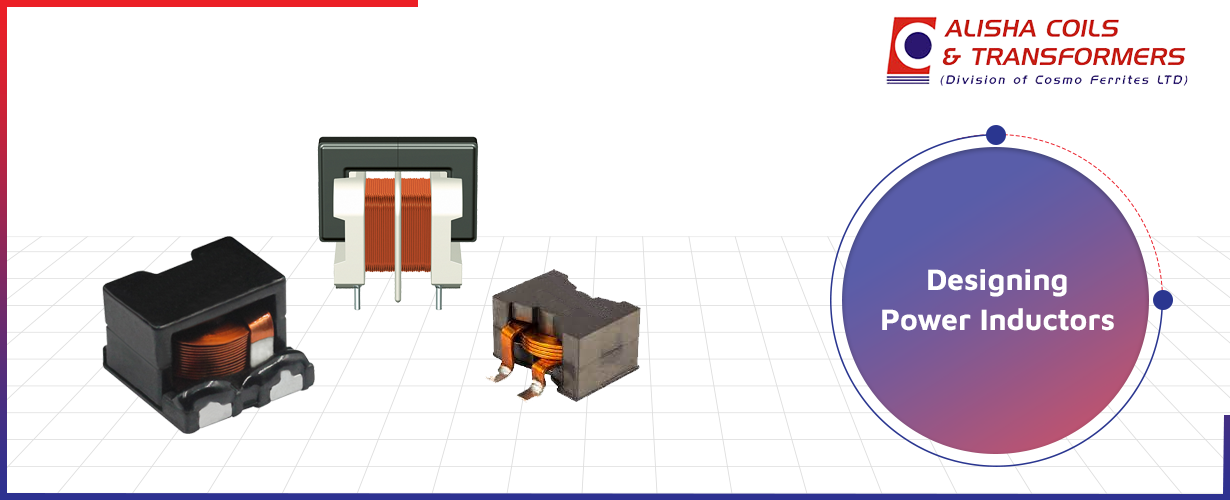##### Request a call back# 6 Tips for Improving the Design of Power InductorsPower Inductors are electronic components used to improve the efficiency of electrical applications. Their design helps to store energy and reduce core losses, which leads to efficient power conversion. But designing power inductors is a complex process. You need to consider a lot of factors before starting with the design.

In this blog, we’re sharing 6 tips that will help you improve the design of power inductors for your applications.

### 1. Switching Frequency

Integrated Circuits are available with a switching frequency from 20 kHz to 2 MHz, which is much better than some regulators.

• To improve the switching frequency levels, you can use materials such as ferrites, powdered iron, and specialty iron alloyed powders.

• If you need switching frequency levels between 100 and 1000 kHz, then you must use both ferrite materials & powdered iron.

• To achieve switching frequency levels higher than 1000 kHz, you can use ferrite materials & specialty iron alloyed powders.

### 2. Inductor Value

Power Inductors are used to minimize power losses in an application. Here, the inductor value is a major factor, as it is connected with ripple current and the unwanted DC current output. Understanding the inverse relationship between the ripple current & inductance value can help you reduce the power losses.

• If the ripple current is smaller, the inductance value is higher.

• If the ripple current is higher, the inductance value is smaller.

Manufacturers provide simulation software along with inductors. The software helps to calculate the two inductor loads—the ripple current load & the DC Current load. But, this calculation can be misunderstood.

• To interpret the calculations, examine the data sheet specifications.

• The nominal current for power inductors is connected to the self-heating with DC current. This self heating of +40°C is the same as the nominal current.

• At the saturation point, the inductance value falls by 10%. However, this isn’t a standard value for data sheet specifications, which results in misinterpretation.

### 4. DC Resistance

DC resistance helps to understand the wire heating losses. Hence, it is an important parameter to reduce the power losses of the power inductor. When you have calculated the required values for inductance L & inductor currents, you need to choose a power inductor of small size, high energy storage density, and low DC resistance. However, small-sized inductors have smaller diameter wires, which increases DC resistance.

• You can get low DC resistance if the size of the inductor is correct.

• If there is higher inductance, you must choose other conductor materials.

### 5. Shielded Inductors

When you use unshielded power inductors, it creates issues when the windings are coupled magnetically with components. To avoid this:

• Use a shielded power inductor for your applications. Make sure that its design doesn’t have circuit boards above or under the component.

• Then, you can place an air gap between these components and avoid magnetic coupling.

• You can use unshielded power inductors if you have low power circuits or less critical applications. With this, you can avoid core losses that occur because of larger core volume. If the dimensions are correct, the core losses are low.

### 6. Output L-C Filter

If you need a low noise output voltage, then an L-C filter at the DC converter output must be used. For this, we recommend you to:

• Select output capacitor

• Calculate the value of inductance

• Choose cut-off frequency at 1/10 to switching regulator frequency

The above tips will help you improve the design of your power inductor & achieve better performance. However, this is just the beginning and there are many more factors that go into the designing of power inductors.If you need more help in the selection of power inductors, you can connect with industry experts at Alisha Coils & Transformers.